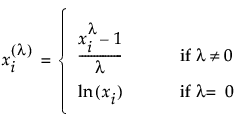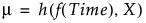Reliability and Survival Methods > Fit Life by X > Launch the Fit Life by X Platform
Publication date: 08/13/2020

# Launch the Fit Life by X Platform

Launch the Fit Life by X platform by selecting Analyze > Reliability and Survival > Fit Life by X. For more information about the options in the Select Columns red triangle menu, see Column Filter Menu in Using JMP.

Figure 4.4 The Fit Life by X Launch WindowThe Fit Life by X launch window contains the following options:

Y, Time to Event

Identifies the time to event (such as the time to failure) or time to censoring. With interval censoring, specify two Y variables, where one Y variable gives the lower limit and the other Y variable gives the upper limit for each unit. For more information about censoring, see Event Plot in the Life Distribution section.

X

Identifies the accelerating factor.

Censor

Identifies censored observations. Select the value that identifies right-censored observations from the Censor Code menu beneath the Select Columns list. The Censor column is used only when one Y is entered.

Freq

Identifies frequencies or observation counts when there are multiple units. If the value is 0 or a positive integer, then the value represents the frequencies or counts of observations for each row when there are multiple units recorded.

By

Identifies a column that creates a report consisting of separate analyses for each level of the variable.

Censor Code

Identifies the value in the Censor column that designates right-censored observations. After a Censor column is selected, JMP attempts to automatically detect the censor code and display it in the box. To change this, you can click the red triangle and select from a list of values. You can also enter a different value in the box. If the Censor column contains a Value Labels column property, the value labels appear in the list of values. Missing values are excluded from the analysis.

Relationship

Identifies the relationship between the event and the accelerating factor. Table 4.1 defines the model for each relationship.

Table 4.1 Models for Relationship Options

Relationship

Model

Arrhenius Celsius

μ = b0 + b1 * 11605 / ( X + 273.15 )

Arrhenius Fahrenheit

μ = b0 + b1 * 11605 / ( ( X + 459.67 ) / 1.8 )

Arrhenius Kelvin

μ = b0 + b1 * 11605 / X

Voltage

μ = b0 + b1 * log( X )

Linear

μ = b0 + b1 * X

Log

μ = b0 + b1 * log( X )

Logit

μ = b0 + b1 * log( X / ( 1 - X ) )

Reciprocal

μ = b0 + b1 / X

Square Root

μ = b0 + b1 * sqrt( X )

Box-Cox

μ = b0 + b1 * BoxCox( X )

Location

means that μ is different for every level of X

Location and Scale

means that μ and σ are both different for every level of X (equivalent to a Life Distribution fit with X as a By variable)

Custom

user-defined μ and σ

If you select Box-Cox, a text edit box appears below the Use Condition option. Use this box to specify a lambda value. The BoxCox( X ) transformation for a specified λ is defined as follows:If you want to use a Custom relationship for your model, see Custom Relationship.

Nested Model Tests

Appends a nonparametric overlay plot, nested model tests, and a multiple probability plot to the report window.

Use Condition

Enables you to enter a value for the explanatory variable, X, of the acceleration factor. You can also set the use condition value after launching the platform using the Set Time Acceleration Use Condition option from the Fit Life by X red triangle menu.

Distribution

Specifies one distribution (Weibull, Lognormal, Loglogistic, Fréchet, SEV, Normal, Logistic, LEV, or Exponential distributions) at a time. Lognormal is the default setting.

Select Confidence Interval Method

Displays the method for computing confidence intervals for the parameters. The default is Wald, but you can select Likelihood instead. The Wald method is an approximation and runs faster. The Likelihood method provides more precise parameters but takes longer to compute.

Note: The Confidence Interval Method preference enables you to select the Likelihood method as the default confidence interval method. You can change this preference in Preferences > Platforms > Fit Life by X.